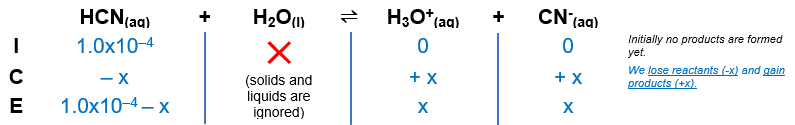# Problem: In a 1.0 x 10-4 M solution of HCN(aq), identify the relative molar amounts of these species. Arrange from most to least. H2OH3O+HCNOH-CN-

###### FREE Expert Solution

HCN is a weak acid. Remember that weak acids partially dissociate in water and that acids donate H+ to the base (water in this case). The dissociation of HCN is as follows:

HCN(aq) + H2O(l)  H3O+(aq) + CN(aq); K= 6.2 × 10–10

From this, we can construct an ICE table. Remember that liquids are ignored in the ICE table.93% (321 ratings)###### Problem Details

In a 1.0 x 10-4 M solution of HCN(aq), identify the relative molar amounts of these species. Arrange from most to least.

H2O
H3O+
HCN
OH-
CN-

Frequently Asked Questions

What scientific concept do you need to know in order to solve this problem?

Our tutors have indicated that to solve this problem you will need to apply the Weak Acids concept. If you need more Weak Acids practice, you can also practice Weak Acids practice problems.

What professor is this problem relevant for?

Based on our data, we think this problem is relevant for Professor Schmidt's class at PURDUE.